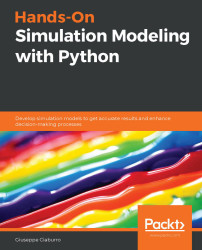•#### Hands-On Simulation Modeling with Python#### Overview of this book

Simulation modeling helps you to create digital prototypes of physical models to analyze how they work and predict their performance in the real world. With this comprehensive guide, you'll understand various computational statistical simulations using Python. Starting with the fundamentals of simulation modeling, you'll understand concepts such as randomness and explore data generating processes, resampling methods, and bootstrapping techniques. You'll then cover key algorithms such as Monte Carlo simulations and Markov decision processes, which are used to develop numerical simulation models, and discover how they can be used to solve real-world problems. As you advance, you'll develop simulation models to help you get accurate results and enhance decision-making processes. Using optimization techniques, you'll learn to modify the performance of a model to improve results and make optimal use of resources. The book will guide you in creating a digital prototype using practical use cases for financial engineering, prototyping project management to improve planning, and simulating physical phenomena using neural networks. By the end of this book, you'll have learned how to construct and deploy simulation models of your own to overcome real-world challenges.
PrefaceSection 1: Getting Started with Numerical SimulationFree Chapter
Chapter 1: Introducing Simulation ModelsChapter 2: Understanding Randomness and Random NumbersChapter 3: Probability and Data Generation ProcessesSection 2: Simulation Modeling Algorithms and TechniquesChapter 4: Exploring Monte Carlo SimulationsChapter 5: Simulation-Based Markov Decision ProcessesChapter 6: Resampling MethodsChapter 7: Using Simulation to Improve and Optimize SystemsSection 3: Real-World ApplicationsChapter 8: Using Simulation Models for Financial EngineeringChapter 9: Simulating Physical Phenomena Using Neural NetworksChapter 10: Modeling and Simulation for Project ManagementChapter 11: What's Next?Other Books You May Enjoy# Understanding the geometric Brownian motion model

The name Brownian comes from the Scottish botanist Robert Brown who, in 1827, observed, under the microscope, how pollen particles suspended in water moved continuously in a random and unpredictable way. In 1905, it was Einstein who gave a molecular interpretation of the phenomenon of movement observed by Brown. He suggested that the motion of the particles was mathematically describable, assuming that the various jumps were due to the random collisions of pollen particles with water molecules.

Today, Brownian motion is, above all, a mathematical tool in the context of probability theory. This mathematical theory has been used to describe an ever-widening set of phenomena, studied by disciplines that are very different from physics. For instance, the prices of financial securities, the spread of heat, animal populations, bacteria, illness, sound, and light are modeled using the same instrument.

Important note

Brownian motion...